# Waves .pdf

### File information

Title: Physics Revision (Light & Sound)
Author: Shoaib Sajid

This PDF 1.4 document has been generated by RAD PDF / RAD PDF 2.25.1.0 - http://www.radpdf.com, and has been sent on pdf-archive.com on 02/02/2015 at 15:46, from IP address 120.63.x.x. The current document download page has been viewed 1309 times.
File size: 535.95 KB (7 pages).
Privacy: public file

### Document preview

Physics Chapter 12: Light

Chapter 12.2: Reflection of Light

Author comment: This is a very BIG chapter!

Chapter 12.1: What is Light?
1.

2.

Light is a form of energy that enables us to see!
a. Our eyes detect light in a range of 7 colours from
red to violet which forms a spectrum.
b. Light travels at 3.0 x 108 metre per second. It can
reach the Earth from the Sun in 8 minutes.
c. The study of the physics of light is known as
optics.

Important terms:
a. Incident Ray: Light ray hitting the reflecting
surface.
b. Reflected Ray: Light ray reflected from the
reflecting surface.
c. Normal: The perpendicular to the reflecting
surface at the point of incidence.
d. Angle of incidence (i): The angle between the
incident ray and the normal.
e. Angle of reflection (r): The angle between the
reflected ray and the normal.

5.

Laws of Reflection: (Words in red and

The Rectilinear Propagation of Light states that light
travels in straight lines.
a. Light cannot bend around corners and can only
travel straight. Proof:

b.
c.

The path in which light travels is known as a light
ray. Arrows are added to indicate the direction of
the light ray.
A beam of light forms a bundle of light rays.

Laser beams
are used in
musical
performance.

d.

3.

4.

Angle of incidence is equal to the angle of
reflection ( i  r )
The incident ray, reflected ray and the
normal at the point of incidence all lie
on the same plane.
6.

Types of Reflection: All surfaces reflect light. The
difference is how smooth the surface is, which will
affect how light is reflected.
a. Regular Reflection: Occurs on smooth surfaces
such as mirrors.
i. The parallel light ray incident on the surface
are reflected in the same direction.
ii. The normal at all points of incidence are
parallel to each other
b. Diffuse Reflection: Occurs on rough surfaces
(e.g. sandpaper)
i. Parallel light rays are reflected in all directions.
ii. Normals are point of incidence are not parallel.

7.

Characteristics of Image formed by plane mirror:
a. Same Size

There are 3 types of beams:
i. Parallel (light rays are straight)
ii. Converging (light rays converge at a point)
iii. Diverging (light rays diverge from a point)

Luminous objects are objects that give out light.
Some examples include the sun, TV, light bulb.
a. Objects that do not give out light are non –
luminous objects.
b. We can see them as that object reflect light from
a luminous object nearby into our eyes.
c. Common mistake: Do not draw light rays
diverging out from the eye!

1

b.
c.
d.
e.

8.

Laterally Inverted
Upright
Virtual
Distance from image from mirror = Distance of
object from mirror.

Applications of Mirrors:
a. Optical Testing (Mirrors can make letters appear
further away, saving space)
b. Blind Corners (for drivers)
c. Periscopes

Chapter 12.3: Refraction at Plane Surfaces
9.

The bending effect of light as it passes from one
transparent material to another is known as
refraction.
a. Refraction is caused by the change in speed of
light.
b. At the boundary of 2 optical media, if there is a
sudden change in the speed of light, it will cause
the path of light to bend.
c. Light travels fastest in air/vaccum.

10. Laws of Refraction: (Words in red and bold are

Snell’s Law: The ratio of the sine of the
angle of incidence to the sine of the angle
sin i
of refraction is constant. (
 constant )
sin r

The incident ray, refracted ray and the
normal at the point of incidence all lie
on the same plane.
11. The refractive index of a medium is a ratio between
the speed of light in air and the speed of light in a
medium.
a.

Therefore,

Speed of light in air
n
Speed of light in medium

sin i
b. Since the constant
 constant , we can
sin r
sin i
say:
 refractive index
sin r
c.

d.

The greater the value of refractive index of a
medium, the greater the bending of light, and the
more denser the material is.
Some common refractive indexes (useful to
Medium
Diamond
Glass
Water

Refractive Index,
2.40
1.48 – 1.96
1.33

Air
e.

f.

1.00

Note the position of the numerator and
denominator. The denser medium is the bottom,
air is always at the top. Snell’s law cannot be used
if neither media is air/vaccum.
Light can be both reflected and refracted at the
boundary of 2 optical medium (to be explored
later)

12. When light travels from a less dense medium to a
denser medium, the ray of light moves towards the
normal.
a. Likewise, when light travels from a denser to a
less dense medium, the ray of light moves away
from the normal.
b. When light enter a medium perpendicularly,
regardless of its density, no deviation of the ray is
observed.
13. The Principle of Reversibility of Light states that light
will follow exactly the same path if its direction of
travel is reversed.
a.

Therefore,

sin r 1

sin i n

14. Phenomena/applications of refraction:
a. Swimming pool appears shallower than it really
is. To find refractive index,

Real depth
n
Apparent depth

Chapter 12.4: Total Internal Reflection
15. Total Internal Reflection occurs when light travels
from an optically denser medium to a less dense
medium.
16. Critical Angle is the angle of incidence in the optically
denser medium where the angle of refraction in the
less dense medium = 90o.
17. 3 important cases of light reflection/refraction.
a. When angle of incidence &lt;
Critical Angle: Normal
Refraction
b. There is still a faint
reflected ray back into the
glass.
a.

b.

When angle of incidence =
Critical Angle: Travels
perpendicular to the
surface (90°)
the refracted ray
gets closer and closer to
the surface of the glass.

2

c.

Can be found by taking

1
c  sin 1  
n
a.
b.
c.

When angle of incidence &gt;
Critical Angle: Total
Internal Reflection.
No light is being refracted
through the glass-to-air
boundary.
Light is totally reflected
back into the glass block.

18. Applications of total internal reflection:
a. Glass prisms
b. Optical fibres: Made up of plastic fibres that
transmit light over long distances through total
internal reflection.
i. An optical fibre has a core of high refractive
index, coated with another material of lower
refractive index.
ii. Light rays entering the fibre will be internally
reflected at the boundary between these 2
refractive materials.

22. Some examples:

Action of a thin converging lens on a parallel beam of light
parallel to the principal axis.

Chapter 12.5: Thin Converging Lens
19. A lens is a clear plastic or glass with curved surfaces.
They are used in magnifying glasses, projectors, etc.
a. There are 2 types of lenses: Converging and
diverging lens.
20. Main features of a converging lens (just briefly know
this terms will do):
a. Optical Centre (C): The midway point between the
lens surface on the principal axis
b. Principal axis: The line passing symmetrically
through the optical centre of the lens
c. Principal focus (F): Point on the principal axis
where rays of light converge after passing
through the lens
d. Focal length (f): Distance between the optical
centre, C and the principal focus F.
e. Focal plane: Plane which passes through F and P.
It is perpendicular to principal axis.
21. There are 3 definite paths of light travelling through a
thin converging lens. Using 2 of these paths, we can
find the position of an image formed by a lens.

Action of a thin converging lens on a parallel beam of light
NOT parallel to the principal axis.

Chapter 12.6: Ray Diagrams for Lenses
Step 1: Setting up the ray diagram.
a. Principle Axis: Draw a horizontal line to represent the
principal axis.
b. Lens: Draw a line perpendicular to the horizontal line
c. Focal Point: Mark a point F on the principle axis on
both sides. The length of each F from the optical
centre should be equal.
Step 2: Placing the object
a. Object: Place the object on the left of the lens by
marking it with an upright arrow.
Step 3: Tracing the light rays and drawing the image
a. Select 2 of the 3 definite paths. Usually the first 2 paths
are selected.
b. The point where the 2 paths intersect on the right side
of the lens is the position of the image.
c. Draw a downward arrow from the principle axis to the
intersection point, and mark it as I. (This denotes that
the image is inverted)
Step 4: Reading the ray diagram
a. An image is inverted if it is below the principle axis.
b. An image is diminished if the distance from it to the
optical centre is lesser than the distance of the object
from the optical centre.

3

c.

An image is real and inverted if it is behind the lens,
else it is virtual and upright.

1.
2.

23. Applications of converging lenses
a. Magnifying glass: It is a thin converging lens that
can be used to make objects look bigger.
b. Camera
c. LCD Projector

3.

1.
2.
3.
4.
5.

Transverse waves are waves that travel perpendicular
to the direction of motion.
Examples of such waves include rope waves and water
waves.
The crest is the highest points of the wave whereas the
trough is the lowest points of the wave.

Longitudinal Waves are waves that travel parallel to
the direction of motion.
Examples are sound wave and pressure waves.
They form compressions and rarefactions.
Compressions are region where the air particles are
close together, creating high pressure.
Rarefactions are areas where the air particles are far
apart, creating low pressure.

Chapter 13.2: Properties of Wave Motion

Physics Chapter 13: Waves
Chapter 13.1: Describing Waves
1.

A wave is made up of periodic motion, which is
motion repeated at regular intervals.
a. A wave is a phenomenon where energy is
transferred through vibrations.
b. One complete motion from one extreme position
to another and back is known as an
oscillation/vibration.
c. Waves can be formed by moving a rope up and
down, using a ripple tank or using a slinky spring.
d. The source of any wave is a vibration or
oscillation.

2.

There are 2 types of wave motion: Transverse and
Longitudinal.

3.

Terms used to describe wave motion:
a. Crests and troughs: The highest and lowest points
of a transverse wave respectively. For
longitudinal waves, they are the regions of
compressions and rarefactions.
b. Phase: Any 2 points are in phase when they move
in the same direction, have the speed and
displacement from rest position. (e.g. 2 crests
and 2 troughs)
c. Wavelength  : The shortest distance between
any 2 points in a wave in phase (e.g. distance
between 2 sucessive crests).
i. SI unit: Metre (m)
d. Amplitude A: It is the maximum displacement
from the rest position; the height of the crest/
depth of a trough from the rest position.
i. SI unit: Metre (m)

4.

2 types of graphs used to plot waves:
a. Displacement-distance graph: Plotting the
displacement of the wave at a certain instant of
time.
b. Displacement-time graph: Used to observe the
displacement of a specific point on a graph over a
4

period of time.
5.

Some wave terms used in the displacement-time
graph:
a. Period (T): Time taken for 1 point on the wave to
complete 1 oscillation, or time taken to produce 1
complete wave. SI unit: second (s)
b. Frequency (f): The number of waves produced per
second. SI unit: Hertz.
i. Formula: f 

1
T

ii. When the frequency of the wave increases, the
period of the wave decreases.
c. Wave speed (v): It is the distance of the wave
moved in 1 second in the medium.
i. SI unit: Metre/second (m/s)
ii. Formula: v 

T

1
iii. From, f  , v  f 
T

7.

Wave refraction: By placing a piece of plastic in the
ripple tank, a region of shallow water is created.
a. The wavelength shortens but the frequency
remains the same as it is determined by the
dipper.
b. Thus by the equation v  f  , the velocity of the
wave will also decrease.

8.
d.

Wavefront: A imaginary
line on a wave joining all
points in the same
phase; usually drawn by
joining the wave crests.
i. Can be of concentric
circles (spherical
dipper), plane straight lines or any other
shape.

Wave reflection: By placing a plastic sheet at an angle
to the incoming waves, the waves will reflect, just like
light waves.

Physics Chapter 14:
Electromagnetic Waves
Chapter 14.1: Electromagnetic Waves

Chapter 13.3: Wave Production and the
Ripple Tank
6.

A ripple tank can be used to generate water waves to
demonstrate wave properties such as reflection and
refraction.

1.

IMPT: Memorise the order of the waves!
a. From longest to shortest wavelength: Radio,
microwaves, infrared, visible, ultraviolet, X-rays,
Gamma Rays
b. Alternatively, Ronald McDonald Is Very Ugly
eXcept Gary!

2.

Properties of electromagnetic waves:

5

a.
b.
c.
d.

e.
f.
g.
h.

3.

Electromagnetic waves are transverse waves.
They are electric and magnetic fields that
oscillate at 90° to each other.
They transfer energy from one place to
another.
They can travel through vacuum (do not require
any medium to travel)
They travel at 3.0 x 108 per second in vacuum.
They will slow down when travelling through
water or glass.
The wave equation v  f  is applicable here too.
They obey the laws of reflection and refraction.
They carry no electric charge (they are neither
positively or negatively charged)
Their frequencies do not change when travelling
from one medium to another. Only their speeds
and wavelength will change.

Physics Chapter 15: Sound
Chapter 15.1: What’s sound?
1.

Sound is a form of energy which enables us to hear.
a. The energy is passed on from one point to
another as a wave.
b. Sound is a form of longitudinal wave.

2.

Sound is produced by vibrating sources placed in a
medium (usually air).
a. Sound cannot travel in vacuum.
b. E.g. of vibrating source: Tuning Fork.

3.

As sound is a longitudinal wave, it travels in a series of
compressions and rarefactions.
a. Compressions: Air molecules are close together,
forms high pressure.
b. Rarefactions: Air molecules are far apart, forms
low pressure.

Uses of electromagnetic waves:
Wave

Microwaves
Infra-red

Uses
Television
Microwave ovens
Communication
system
Thermal imaging
Remote controls

Dangers

Chapter 15.2: Transmission of Sound

None
4.

Sound waves need a medium in order to travel.
a. Expt: The bell jar experiment; a bell is placed in a
jar where the air is slowly sucked out. The sound
gradually faints till there is no air inside.

5.

Approximate speed of sound in various mediums (use
this to check answers – ensure not too far off):
a. Air: 300 – 340m/s (Measured by firing pistol and
calculating time difference between the 2
sounds). Source of error: Human reaction time.
Improve result: Repeat expt, take avg. value and
exchange positions of observers.
b. Water: 1500 m/s
c. Glass: 5000 m/s

Internal heating
of body tissue
Burns skin

Optic fibres
Seeing!

Strong light
causes damage
to vision.

Washing powder
(whiter than white)
Security marking

Skin cancer and
blindness

X rays

Taking images of
the skeleton

Mutations in
cells and severe
burns to the
skin.

Chapter 15.3: Reflection of Sound

Gamma
Rays

Cancer treatment
Sterilisation of
equipment

Cancers and cell
mutation

6.

Light

Ultra-violet

Just memorise some of these…

Echos refer to the repetition of a sound resulting from
reflection of the sound waves.
a. An echo is formed when a sound is reflected off
hard, flat surfaces such as a wall/cliff.
b. Reverberation occurs when the surface is too
close, causing any reflected sound to follow
closely behind the direct sound and prolonging
the original sound.

Chapter 15.4: Ultrasound
7. The range of frequencies which a person can hear
is known as the range of audibility.
a. Human: Between 20 Hz and 20 kHz1
b. Dog: &lt;20 kHz
c. Bats: Between 10 kHz and 120 kHz.
8. Ultrasound is the sounds with frequencies above
the upper limit of the human range of audibility.

6

Its small wavelength means less diffraction and
the echo formed is more precise in direction.
9. Applications for ultrasound include:
a. Determining depth of seabed
b. Locating sunken ships / shoals of fish
c. Cleaning small dirt from jewellery.
d. Quality control (checking for cracks) in
concrete
e. Medical applications (development of foetus)

Chapter 15.5: Pitch and Loudness
Loudness and Pitch

10. Loudness is a factor distinguishing between various
sounds.
a. The larger the amplitude of vibration, the louder
the sound
b. Sound is measured by decibels (dB).
11. Pitch is a factor distinguishing various sounds
a. The higher the frequency of a note, the higher
the pitch
b. Pitch is measured in hertz (Hz).

7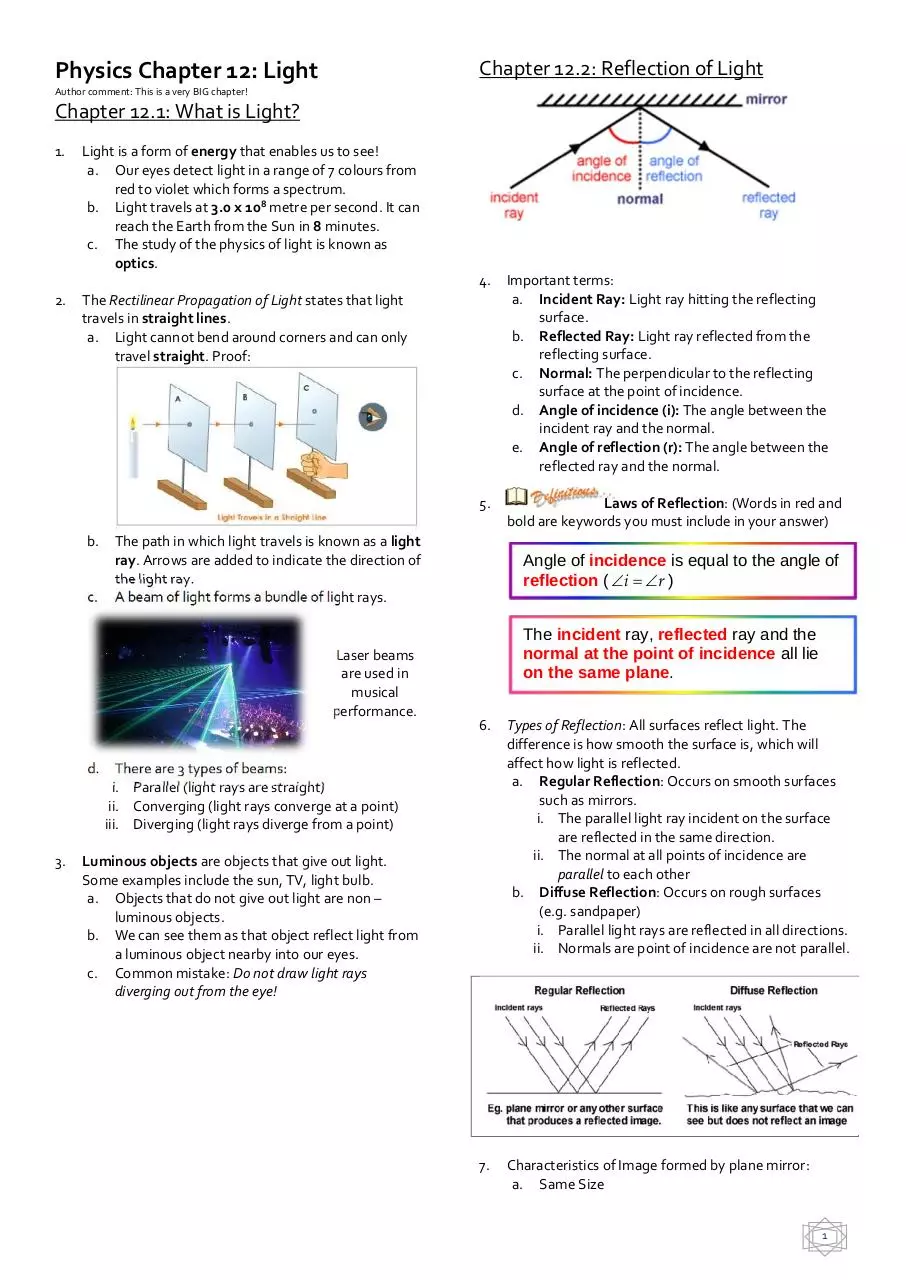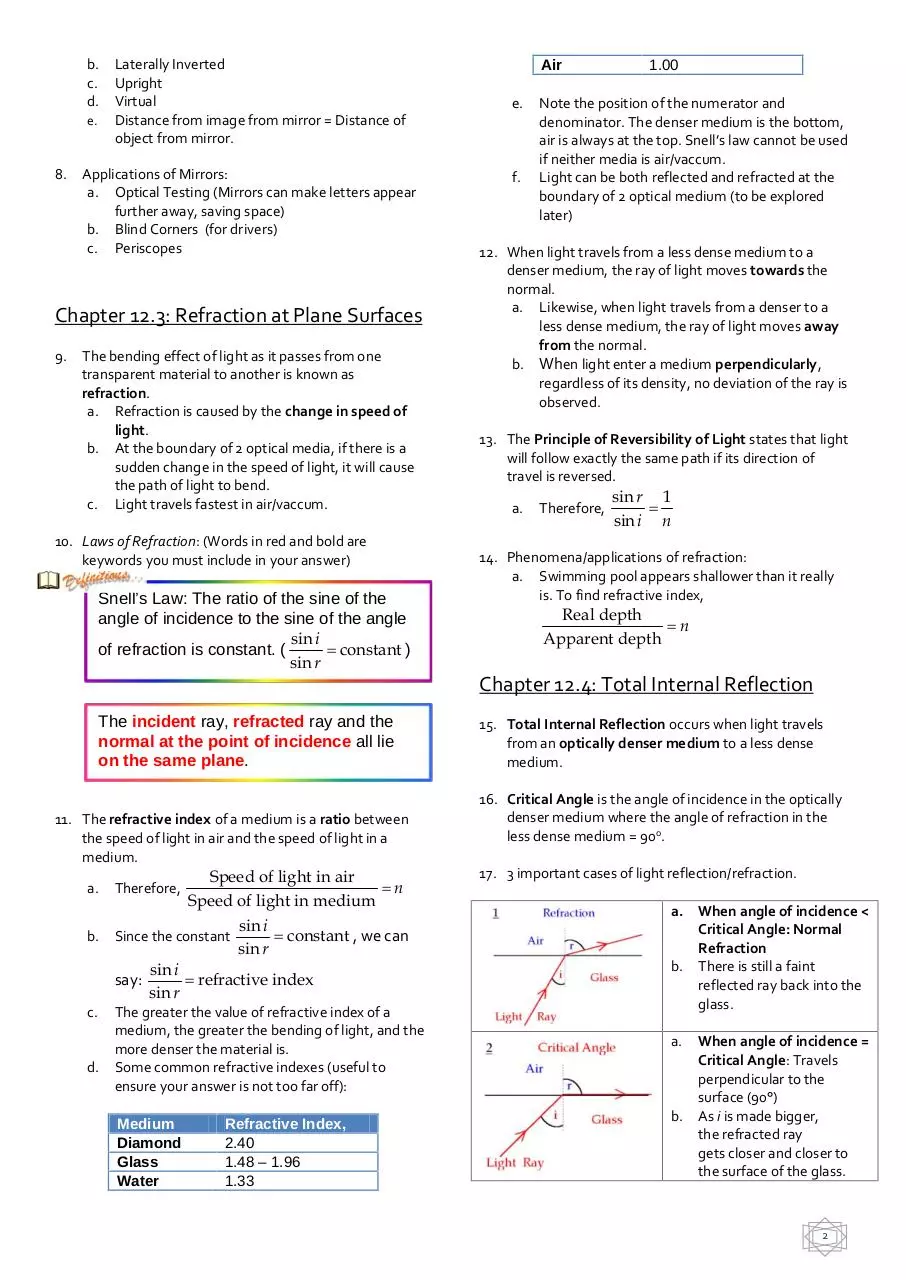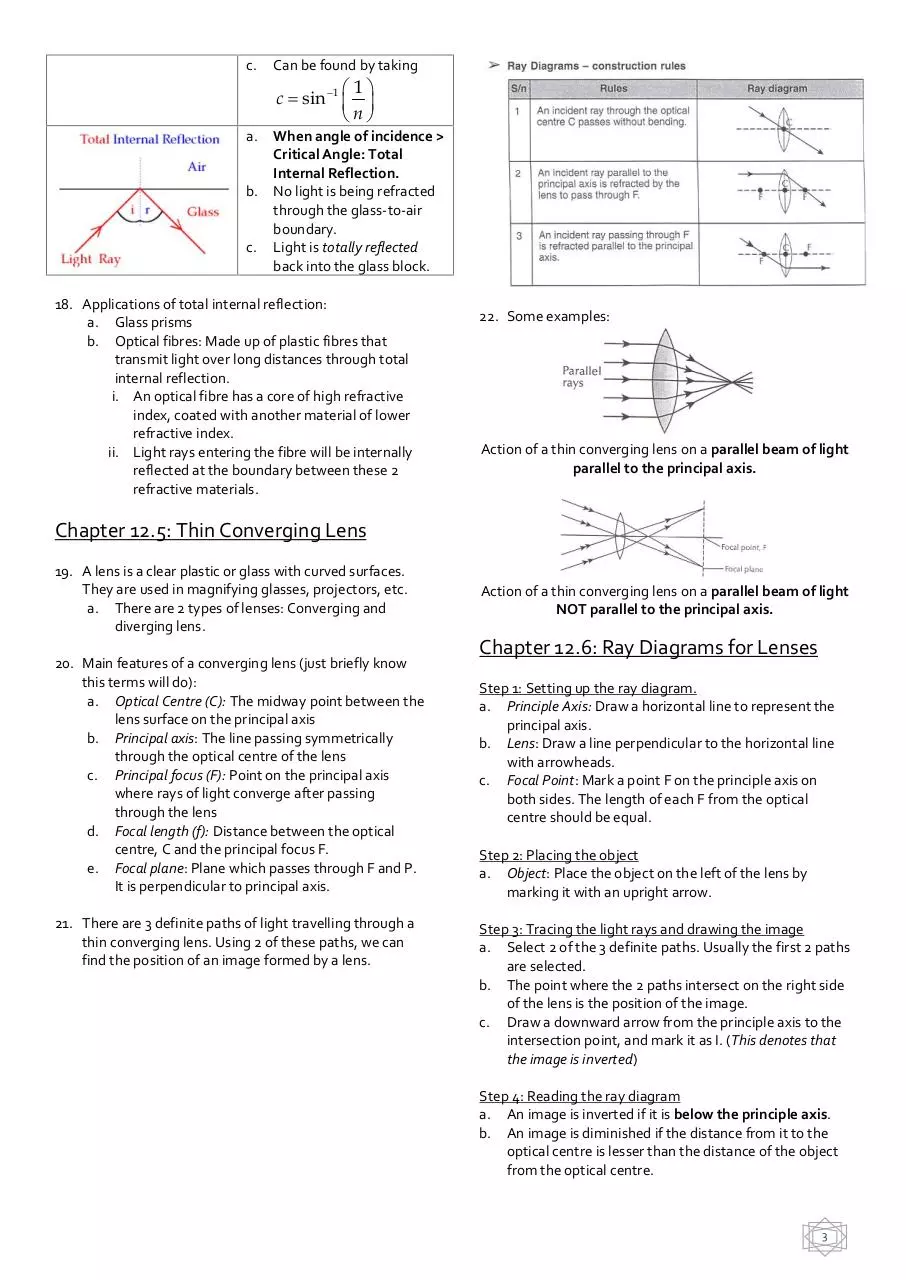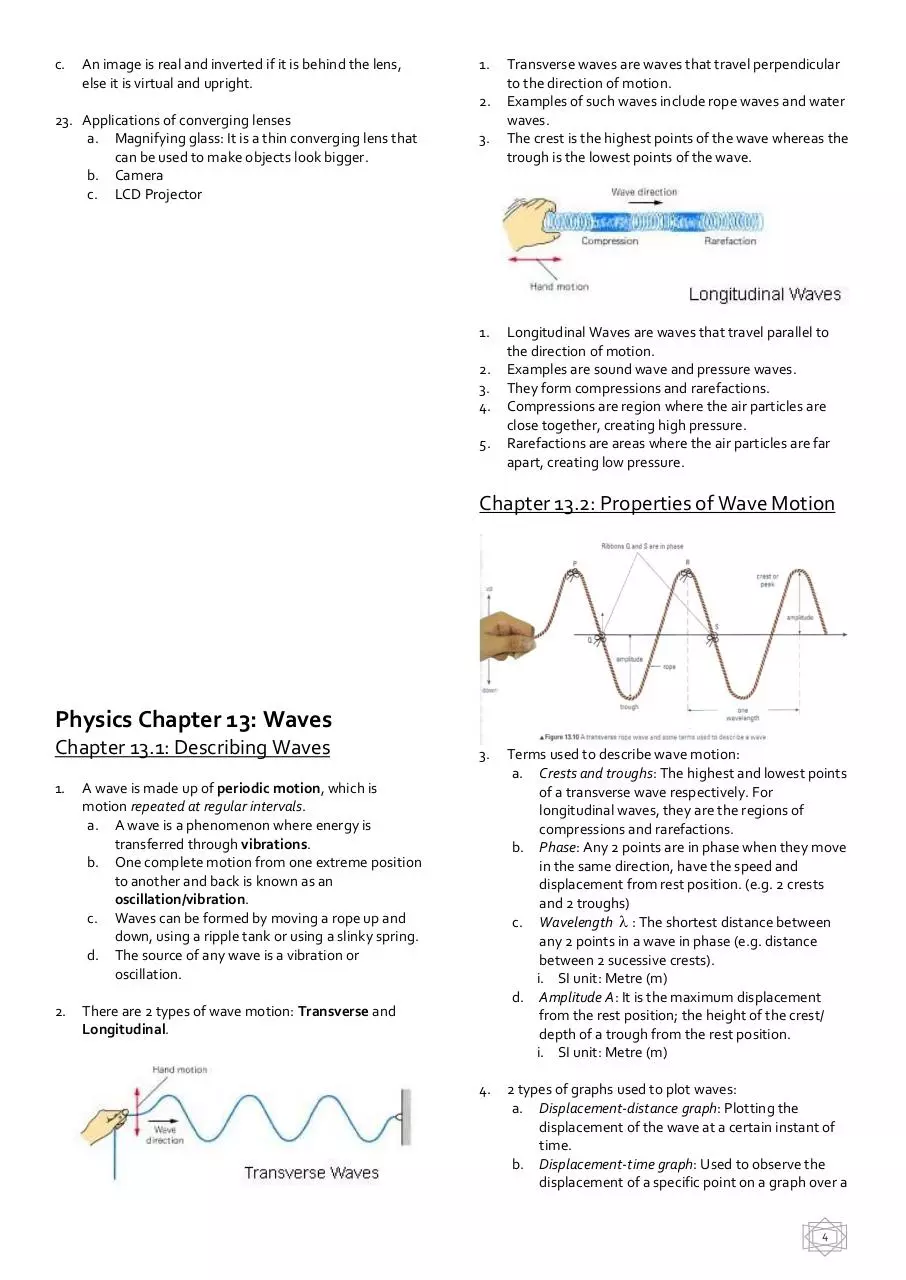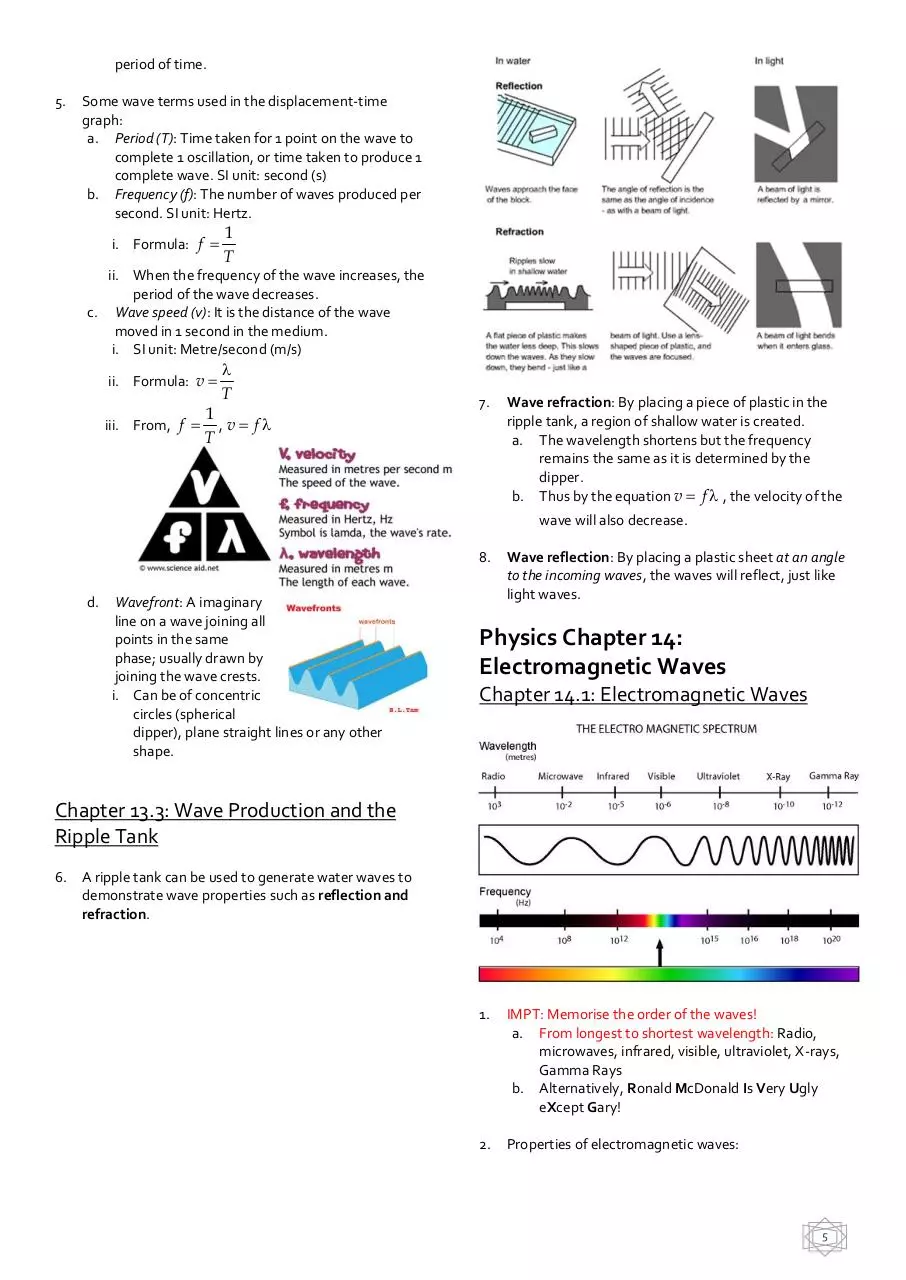Waves.pdf (PDF, 535.95 KB)

### Share on social networks

#### HTML Code

Copy the following HTML code to share your document on a Website or Blog